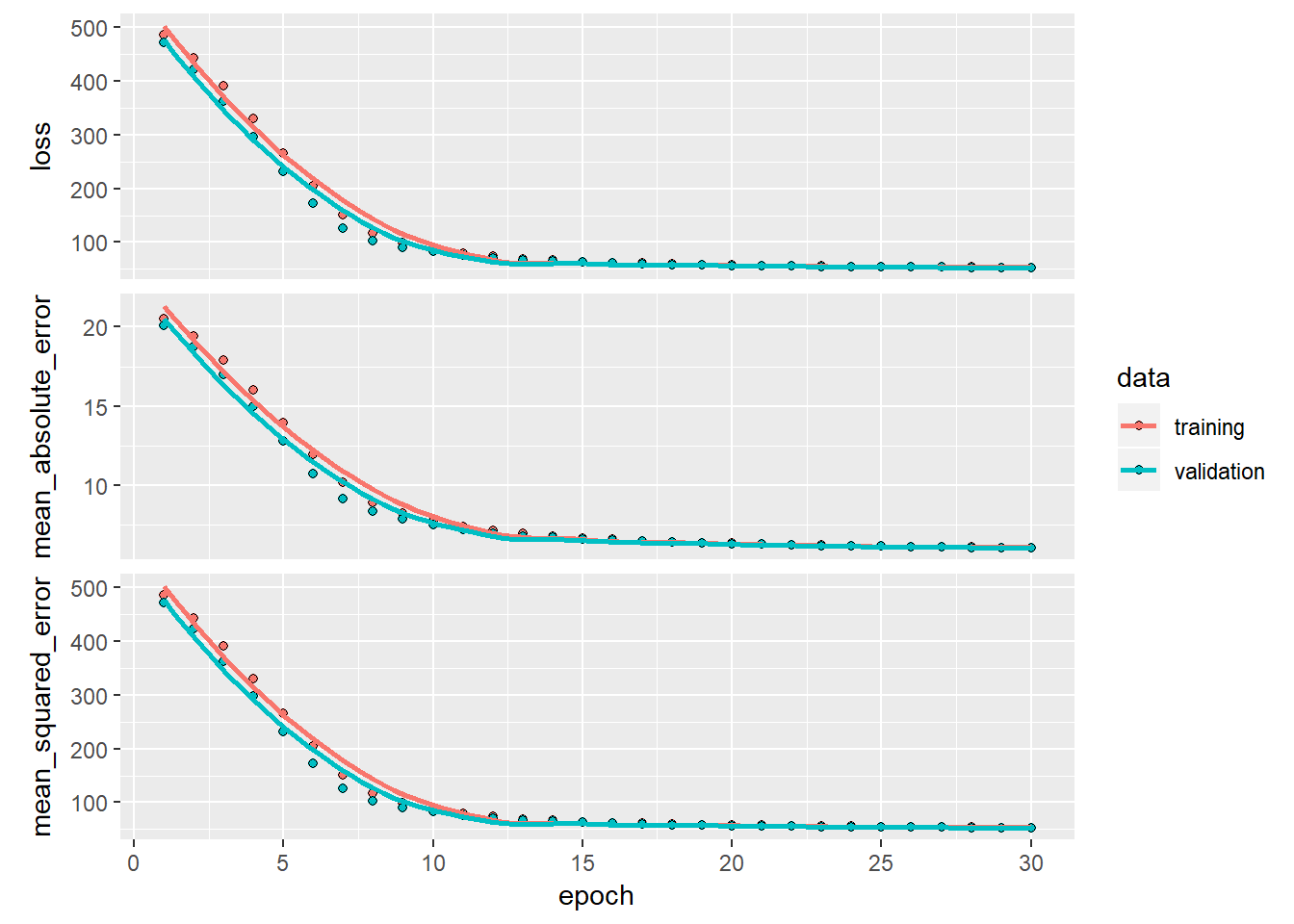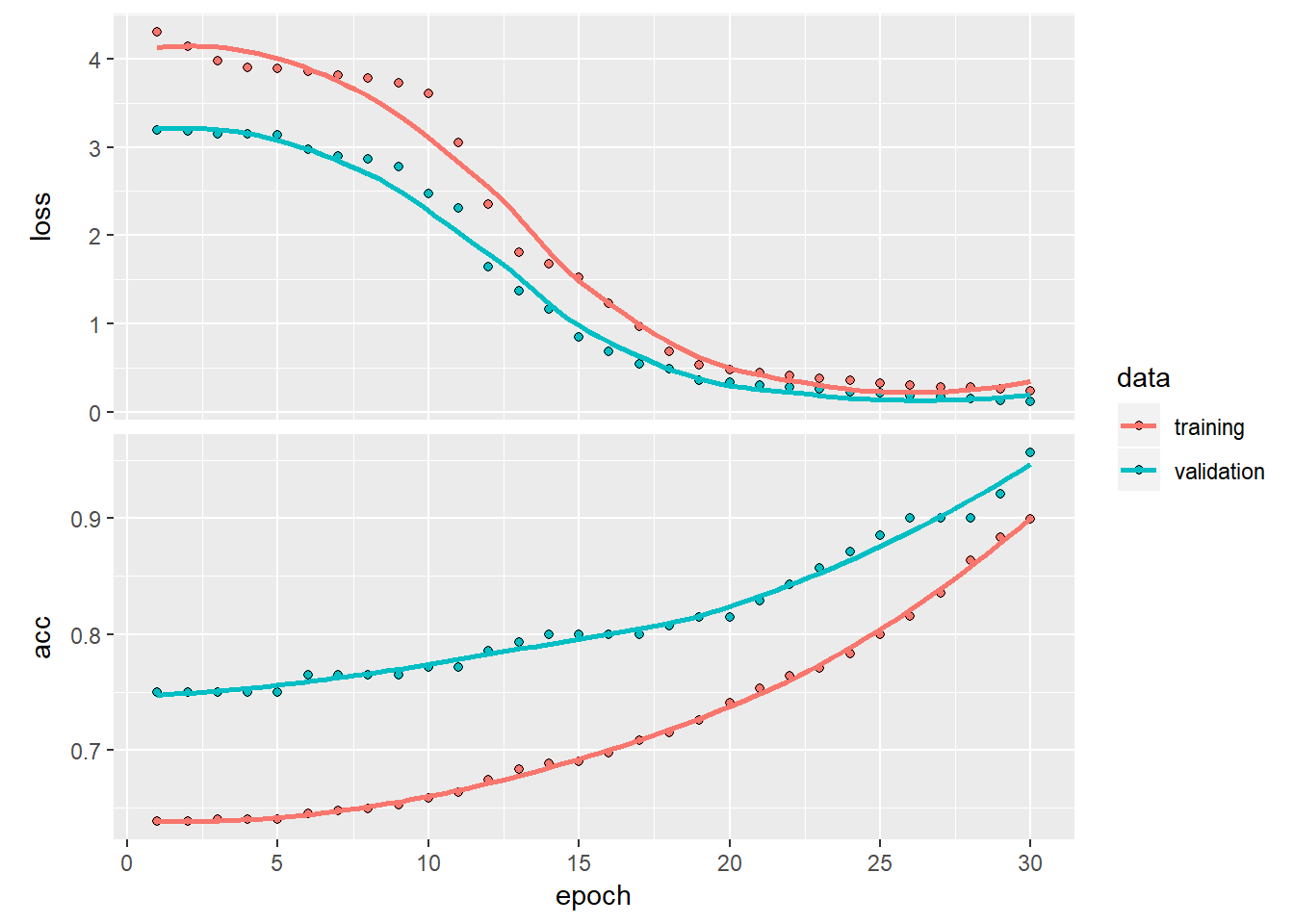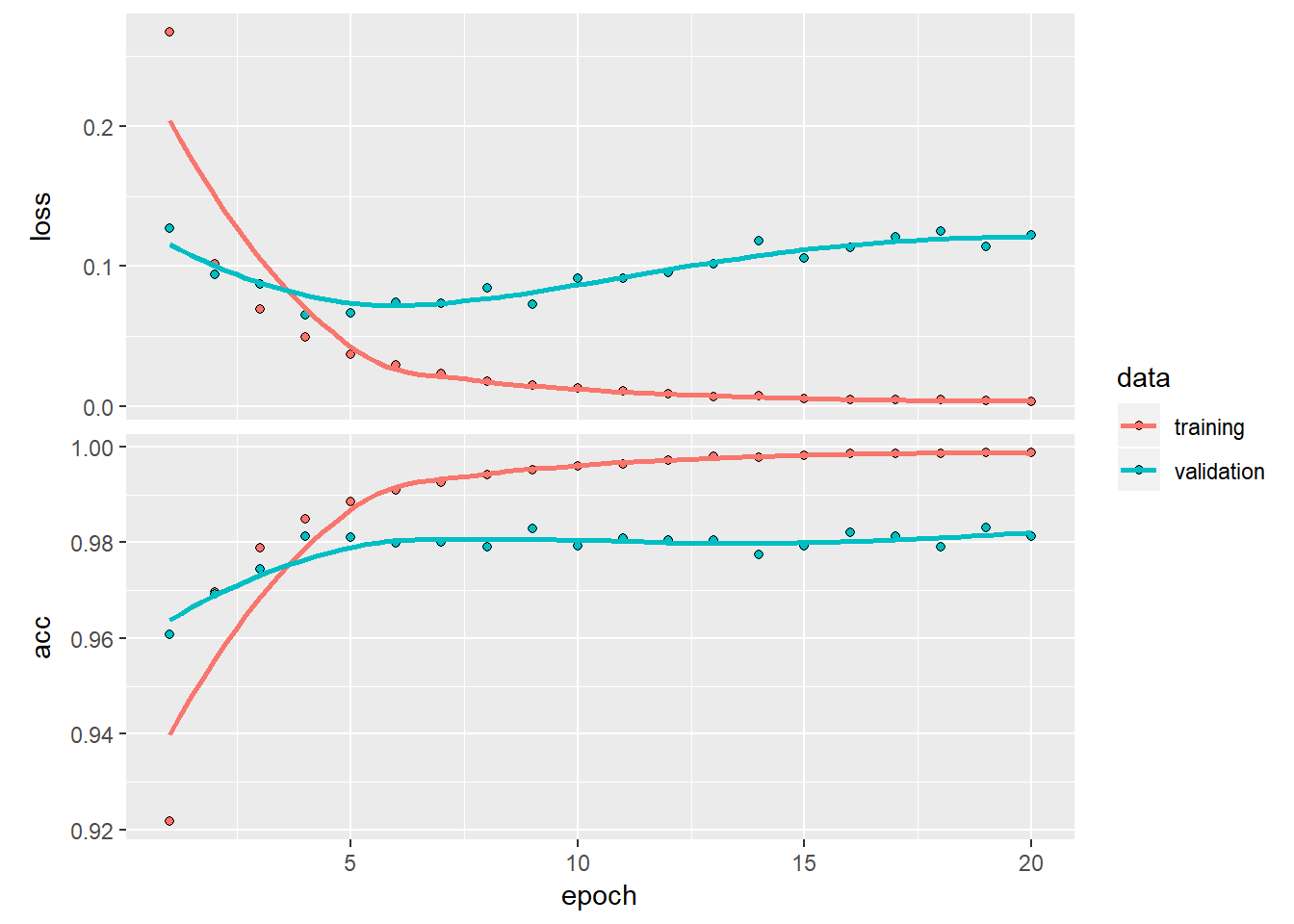## Introduction

In this post I show how to get started with Tensorflow and Keras in R.

# Install Tensorflow in RStudio
#install_tensorflow()
# Install Keras
#install_packages("keras")
library(tensorflow)
## Warning: package 'tensorflow' was built under R version 3.6.1
library(keras)
## Warning: package 'keras' was built under R version 3.6.1
library(dplyr)
##
## Attaching package: 'dplyr'
## The following objects are masked from 'package:stats':
##
##     filter, lag
## The following objects are masked from 'package:base':
##
##     intersect, setdiff, setequal, union
library(dummies)
## dummies-1.5.6 provided by Decision Patterns
library(tensorflow)
library(keras)

## Multivariate regression

This code performs multivariate regression using Tensorflow and keras on the advent of Parkinson disease through sound recordings see Parkinson Speech Dataset with Multiple Types of Sound Recordings Data Set. The clinician’s motorUPDRS score has to be predicted from the set of features.

# Download the Parkinson's data from UCI Machine Learning repository

# Set the column names
names(dataset) <- c("subject","age", "sex", "test_time","motor_UPDRS","total_UPDRS","Jitter","Jitter.Abs",
"Jitter.RAP","Jitter.PPQ5","Jitter.DDP","Shimmer", "Shimmer.dB", "Shimmer.APQ3",
"Shimmer.APQ5","Shimmer.APQ11","Shimmer.DDA", "NHR","HNR", "RPDE", "DFA","PPE")

# Remove the column 'subject' as it is not relevant to analysis
dataset1 <- subset(dataset, select = -c(subject))

# Make the column 'sex' as a factor for using dummies
dataset1$sex=as.factor(dataset1$sex)
# Add dummy variables for categorical cariable 'sex'
dataset2 <- dummy.data.frame(dataset1, sep = ".")
## Warning in model.matrix.default(~x - 1, model.frame(~x - 1), contrasts =
## FALSE): non-list contrasts argument ignored
dataset3 <- na.omit(dataset2)

### Split the data as training and test in 80/20

## Split data 80% training and 20% test
sample_size <- floor(0.8 * nrow(dataset3))

## set the seed to make your partition reproducible
set.seed(12)
train_index <- sample(seq_len(nrow(dataset3)), size = sample_size)

train_dataset <- dataset3[train_index, ]
test_dataset <- dataset3[-train_index, ]

train_data <- train_dataset %>% select(sex.0,sex.1,age, test_time,Jitter,Jitter.Abs,Jitter.PPQ5,Jitter.DDP,
Shimmer, Shimmer.dB,Shimmer.APQ3,Shimmer.APQ11,
Shimmer.DDA,NHR,HNR,RPDE,DFA,PPE)

train_labels <- select(train_dataset,motor_UPDRS)
test_data <- test_dataset %>% select(sex.0,sex.1,age, test_time,Jitter,Jitter.Abs,Jitter.PPQ5,Jitter.DDP,
Shimmer, Shimmer.dB,Shimmer.APQ3,Shimmer.APQ11,
Shimmer.DDA,NHR,HNR,RPDE,DFA,PPE)
test_labels <- select(test_dataset,motor_UPDRS)

## Normalize the data

 # Normalize the data by subtracting the mean and dividing by the standard deviation
normalize<-function(x) {
y<-(x - mean(x)) / sd(x)
return(y)
}

normalized_train_data <-apply(train_data,2,normalize)
# Convert to matrix
train_labels <- as.matrix(train_labels)
normalized_test_data <- apply(test_data,2,normalize)
test_labels <- as.matrix(test_labels)

### Create the Deep Learning Model

model <- keras_model_sequential()
model %>%
layer_dense(units = 6, activation = 'relu', input_shape = dim(normalized_train_data)) %>%
layer_dense(units = 9, activation = 'relu') %>%
layer_dense(units = 6, activation = 'relu') %>%
layer_dense(units = 1)

# Set the metrics required to be Mean Absolute Error and Mean Squared Error.For regression, the loss is
# mean_squared_error
model %>% compile(
loss = 'mean_squared_error',
optimizer = optimizer_rmsprop(),
metrics = c('mean_absolute_error','mean_squared_error')
)

# Fit the model
# Use the test data for validation
history <- model %>% fit(
normalized_train_data, train_labels,
epochs = 30, batch_size = 128,
validation_data = list(normalized_test_data,test_labels)
)

### Plot mean squared error, mean absolute error and loss for training data and test data

plot(history)
## Registered S3 methods overwritten by 'ggplot2':
##   method         from
##   [.quosures     rlang
##   c.quosures     rlang
##   print.quosures rlang## Observation

It can be seen that the mean absolute error is on an average about +/- 4.0. The validation error also is about the same. This can be reduced by playing around with the hyperparamaters and increasing the number of iterations

## Classification

This is a simple binary classification problem from UCI Machine Learning repository and deals with data on Breast cancer from the Univ. of Wisconsin Breast Cancer Wisconsin (Diagnostic) Data Set

# Read the data for Breast cancer (Wisconsin)

# Rename the columns
"barenuclei","chromatin","normalnucleoli","mitoses","class")

# Remove the columns id and class
dataset1 <- subset(dataset, select = -c(id, class))
dataset2 <- na.omit(dataset1)

# Convert the column to numeric
dataset2$barenuclei <- as.numeric(dataset2$barenuclei)

## Normalize the data

train_data <-apply(dataset2,2,normalize)
train_labels <- as.matrix(select(dataset,class))

# Set the target variables as 0 or 1 as it binary classification
train_labels[train_labels==2,]=0
train_labels[train_labels==4,]=1

### Create the Deep Learning model

model <- keras_model_sequential()
model %>%
layer_dense(units = 6, activation = 'relu', input_shape = dim(train_data)) %>%
layer_dense(units = 9, activation = 'relu') %>%
layer_dense(units = 6, activation = 'relu') %>%
layer_dense(units = 1)

# Since this is a binary classification we use binary cross entropy
model %>% compile(
loss = 'binary_crossentropy',
optimizer = optimizer_rmsprop(),
metrics = c('accuracy')  # Metrics is accuracy
)

### Fit the model. Use 20% of data for validation

history <- model %>% fit(
train_data, train_labels,
epochs = 30, batch_size = 128,
validation_split = 0.2
)

### Plot the accuracy and loss for training and validation data

plot(history)## MNIST

The following code uses Tensorflow to learn MNIST’s handwritten digits ### Load MNIST data

mnist <- dataset_mnist()
x_train <- mnist$train$x
y_train <- mnist$train$y
x_test <- mnist$test$x
y_test <- mnist$test$y

### Reshape and rescale

# Reshape the array
x_train <- array_reshape(x_train, c(nrow(x_train), 784))
x_test <- array_reshape(x_test, c(nrow(x_test), 784))
# Rescale
x_train <- x_train / 255
x_test <- x_test / 255

### Convert out put to One Hot encoded format

y_train <- to_categorical(y_train, 10)
y_test <- to_categorical(y_test, 10)

### Fit the model

Use the softmax activation for recognizing 10 digits and categorical cross entropy for loss

model <- keras_model_sequential()
model %>%
layer_dense(units = 256, activation = 'relu', input_shape = c(784)) %>%
layer_dense(units = 128, activation = 'relu') %>%
layer_dense(units = 10, activation = 'softmax') # Use softmax

model %>% compile(
loss = 'categorical_crossentropy',
optimizer = optimizer_rmsprop(),
metrics = c('accuracy')
)

### Fit the model

Note: A smaller number of epochs has been used. For better performance increase number of epochs

history <- model %>% fit(
x_train, y_train,
epochs = 20, batch_size = 128,
validation_data = list(x_test,y_test)
)

### Plot the accuracy and loss for training and test data

plot(history)`